# Utah Data Competition 2014¶

### Jed Ludlow¶

jedludlow.com

In :
%matplotlib inline

In :
import matplotlib.pyplot as plt
import seaborn as sns
import numpy as np
import time


Setup seaborn to use slightly larger fonts

In :
sns.set_context("talk")


# Introduction¶

This notebook documents two of the modeling approaches I pursued as part of the 2014 Utah Data Competition. The stated problem focuses on the prediction of wafer yields in a simulated semiconductor fab. More details about the problem are available elsewhere.

# Special Scoring Function¶

A key aspect to the problem is a special scoring function that penalizes undercall by ten times.

In :
def score_fun(y_hat, y, clamp=True):
"""
Scoring function with special penalty for undercall.

y_hat and y are 1-D arrays.

"""
if clamp:
y_hat = np.clip(y_hat, 0.0, 600.0)

err = y_hat - y

score = (
np.sum(np.abs(err[err < 0.0])) * 10.0 +
np.sum(np.abs(err[err >= 0.0]))
) / len(err)

return score


# Methods¶

Most of my efforts were focused on two model types: Ridge regression and Kalman filtering.

## Ridge Regression with Special Penalty for Undercall¶

Here is a modified Ridge regression model that accounts for the special undercall penalty. It also adds provision for weighting. Consider the training set $X$ to contain $m$ observations of the $n$ context inputs. Assume a linear model of the form $\hat{y} = X \theta$, where $\theta$ is the vector of die loss estimates at each context.

For the $i$-th observation,

### Residuals¶

$$r^{(i)} = x_1^{(i)} \theta_1 + \dots + x_n^{(i)} \theta_n- y^{(i)}$$

### Penalties¶

$$k^{(i)} = \left\{ \begin{array}{l l} 1 & \quad \textrm{if}\quad r^{(i)} \ge 0 \\ -10 & \quad \textrm{otherwise} \end{array} \right.$$

### Weights¶

$$w^{(i)} = \alpha^{m - i}$$

### Cost Function¶

$$J(\theta) = \frac{1}{m} \left[ \sum_{i=1}^{m} w^{(i)} k^{(i)} r^{(i)} + \frac{\lambda}{2} \sum_{j=1}^{n} \theta_j^2 \right]$$

$$\frac{\partial J}{\partial \theta_j} = \frac{1}{m} \left[ \sum_{i=1}^{m} w^{(i)} k^{(i)} x_j^{(i)} \theta_j + \lambda \theta_j \right], \quad j=1 \ldots n$$

### Ridge Regression Class Implementation¶

Create a simple class that mirrors the interface of similar models from sklearn. Make use of standard SciPy funciton minimizers in the model fitting method.

In :
from scipy.optimize import minimize

class RidgeRegressSpecialPenalty(object):
"""
Ridge regression model with special penalty function.

"""
opts = {'maxiter': 20000}

def __init__(self, lam=1.0, tol=1e-3, solver='L-BFGS-B'):
self.lam = lam
self.tol = tol
self.solver = solver

def fit(self, X, y, theta_guess, w=None, bounds=None):
self.X = X.copy()
self.y = y.copy()
if w is not None:
self.w = w
else:
self.w = np.ones_like(y)
self.m = len(y)

ret = minimize(
self.cost_fun, theta_guess, jac=True,
method=self.solver, tol=self.tol, options=self.opts,
bounds=bounds
)
self.theta = ret.x
return ret

def predict(self, X):
y_hat = X.dot(self.theta)
return y_hat

def cost_fun(self, theta):
# Initialize penalty array
k = np.ones_like(self.y)

# Compute residuals and penalize undercalls
h_t = self.X.dot(theta)
res = h_t - self.y
k[res < 0] *= -10.0

# Weights
k = k * self.w

# Compute cost
J = (
(1.0 / self.m) * np.sum(k * res) +
(self.lam / (2.0 * self.m)) * np.sum(theta**2)
)

(1.0 / self.m) * np.dot(self.X.T, k) +
(self.lam / self.m) * theta
)



## Approximate Kalman Filter¶

A Kalman filter is a special type of recursive Bayesian filter, used to estimate the underlying state of a system from a time series of measurements that are some linear combination of those states.

For the $k$-th time step,

### Predict¶

\begin{align} \hat{\theta}_k^- & = \hat{\theta}_{k-1} \\ P_k^- & = P_{k-1} + Q \end{align}

### Correct¶

\begin{align} K_k & = P_k^- H_k^T (H_k P_k^- H_k^T + R)^{-1} \\ \hat{\theta_k} & = \hat{\theta}_k^- + K_k(y_k - H \hat{\theta}_k^-) \\ P_k & = (I - K_k H) P_k^- \end{align}
• To minimize training time, the Kalman covariance matrix $P$ was approximated by considering only the diagonal terms of the matrix. Instead of a 2000x2000 matrix, the covariance is stored as a 2000 element vector. This approach has been applied previously with some success.

• How well can we expect to estimate 2000 context states when we only observe total die loss at each measurement?

# Preliminary Data Set¶

Let's examine how well each of these models performs on the preliminary data sets.

In :
with np.load("prelim_files.npz") as data:
X = data['X']
Y = data['Y']
X_val = data['X_val']
Y_val = data['Y_val']
del data


## Ridge Regression¶

Rough grid search using learning decay rate $\alpha$ and Ridge parameter $\lambda$ as tuning parameters on the preliminary validation set produces a best score of 222.1 against the validation set.

In :
# Uncomment, augment these for grid search
# rates = [1.0, .9999, .999, .99, .9, .8]
# lambdas = [0.0001, 0.0005, 0.001, 0.005, 0.01, 0.1,]

rates = [0.999,]
lambdas = [0.001,]
results = []
for rate in rates:
for lam in lambdas:
t0 = time.clock()

# Initialize starting guess, set weights, set bounds
guess = np.ones(X.shape)
weights = np.flipud(rate ** np.arange(len(Y)))
bounds = [(0.0, 600.0)] * len(guess)

# Initialize model, fit, predict
ridge = RidgeRegressSpecialPenalty(lam=lam, tol=1e-6)
ret = ridge.fit(X, Y, guess, weights, bounds)
Y_pred_ridge = ridge.predict(X_val)

# Score against validation set
score = score_fun(Y_pred_ridge, Y_val, clamp=True)

# Store results
results.append((ret, score, rate, lam, ridge))
print "Status:", ret.status, "Sec:", (time.clock() - t0), "Score:", score, "Rate:", rate, "Lambda:", lam

Status: 0 Sec: 38.161585 Score: 222.07508588 Rate: 0.999 Lambda: 0.001


### Ridge Model Coefficients¶

In :
plt.plot(ridge.theta)
plt.xlabel("Context Number $j$")
_ = plt.ylabel(r"$\theta_j$")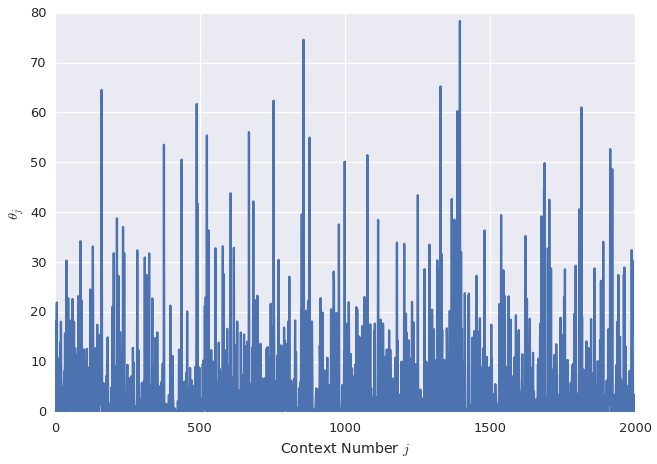### Ridge Predicted Die Loss for Preliminary Validation Data Set¶

In :
plt.plot(Y_pred_ridge)
plt.xlabel("Validation Wafer")
_ = plt.ylabel("Predicted Die Loss")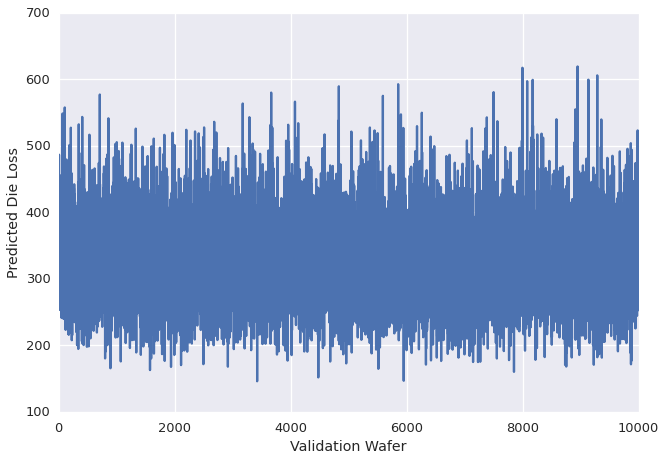### Ridge Scatter Plot for Preliminary Validation Data Set¶

In :
fig = plt.figure()
plt.scatter(Y_val, Y_pred_ridge, marker='.', color=sns.color_palette())
plt.axis([0, 600, 0, 600])
plt.xlabel("Actual Die Loss")
_ = plt.ylabel("Predicted Die Loss")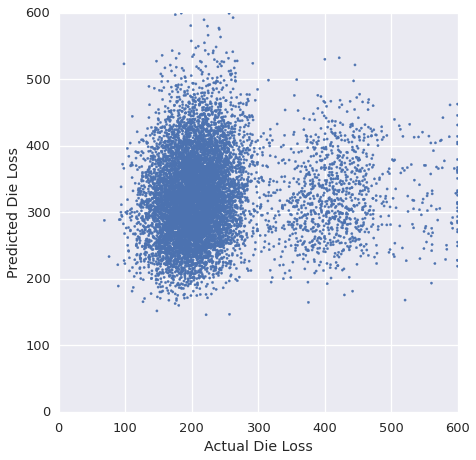## Approximate Kalman Filter¶

Rough grid search using $Q$ (process covariance), $R$ (measurement covariance), and a constant offset as tuning parameters yields a best score of 208.8, outperforming the Ridge model.

In :
Qs = [5.99484250e-04,]
Rs = [2.15443469e+02,]
offsets = [1.0,]

# Uncomment for grid search
# Qs = np.logspace(-5.0, -3.0, 10)
# Rs = np.logspace(1.0, 3, 10)
# offsets = [0.0, 0.5, 1.0, 1.5, 2.0,]

H = X
results = []
for _, _Q in np.ndenumerate(Qs):
for _, R in np.ndenumerate(Rs):
for offset in offsets:

# Initialize
Q = _Q * np.ones(2000)
theta_hats = np.zeros((9999, 2000))
theta_hat_minus = np.zeros(2000)
K = np.zeros(2000)
theta_hat_0 = np.zeros(2000)
P = 1.0 * np.ones(2000)

# Run the filter for each time step
for k in range(len(Y)):
# time update (predict)
if k != 0:
theta_hat_minus = theta_hats[k-1]
else:
theta_hat_minus = theta_hat_0

P_minus = P + Q

# measurement update (correct)
K = P_minus * H[k] / (P_minus.dot(H[k]) + R)
res = Y[k] - theta_hat_minus.dot(H[k])
theta_hats[k] = np.clip(theta_hat_minus + K * res, 0.0, 600.0)
P = (1.0 - K * H[k]) * P_minus

theta_offset = offset + theta_hats[-1]
Y_pred_kalman = X_val.dot(theta_offset)
score = score_fun(Y_pred_kalman, Y_val)
results.append([score, _Q, R, offset])
print "Score", score, "Q", _Q, "R", R, "Offset", offset

results = np.array(results)

Score 208.803322581 Q 0.00059948425 R 215.443469 Offset 1.0


### Kalman Model Coefficients¶

In :
plt.plot(theta_offset)
plt.xlabel("Context Number $j$")
_ = plt.ylabel(r"$\theta_j$")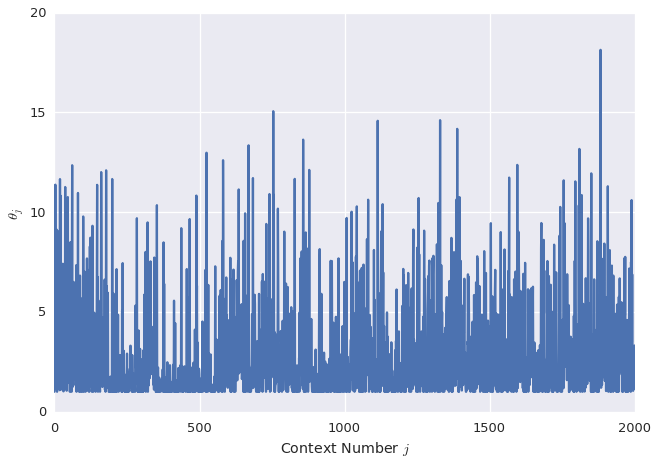### Kalman Predicted Die Loss for Preliminary Validation Data Set¶

In :
plt.plot(Y_pred_kalman)
plt.xlabel("Validation Wafer")
_ = plt.ylabel("Predicted Die Loss")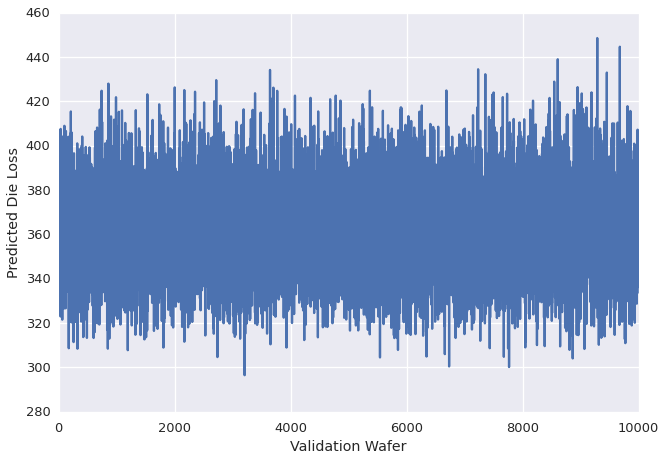### Kalman Scatter Plot for Preliminary Validation Set¶

In :
fig = plt.figure()
plt.scatter(Y_val, Y_pred_kalman, marker='.', color=sns.color_palette())
plt.axis([0, 600, 0, 600])
plt.xlabel("Actual Die Loss")
_ = plt.ylabel("Predicted Die Loss")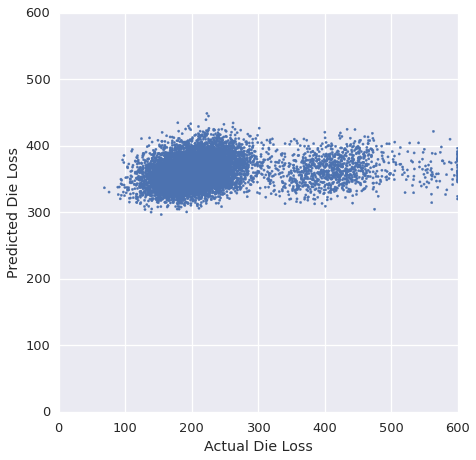## Compare Ridge and Kalman Model Predictions¶

In :
fig = plt.figure()
plt.scatter(Y_pred_ridge, Y_pred_kalman, marker='.', color=sns.color_palette())
plt.axis([0, 600, 0, 600])
plt.xlabel("Ridge Predicted Loss")
_ = plt.ylabel("Kalman Predicted Loss")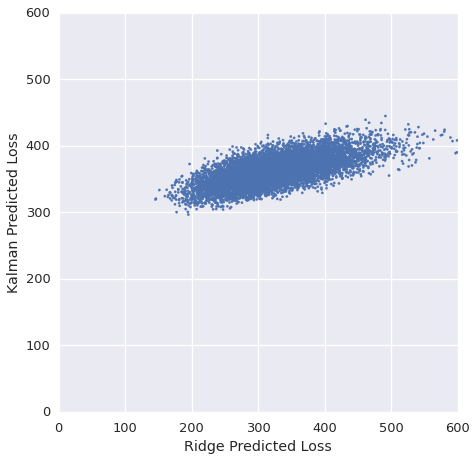## Compare Ridge and Kalman Model Coefficients¶

In :
fig = plt.figure()
plt.plot(ridge.theta)
plt.ylabel(r"Ridge $\theta_j$")
plt.plot(theta_offset)
plt.xlabel("Context Number $j$")
_ = plt.ylabel(r"Kalman $\theta_j$")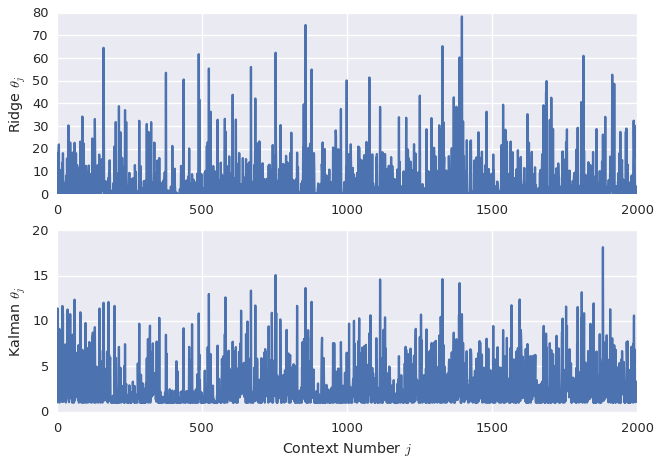# Final Data Set¶

Examine performance on the final data sets. The process dynamics are signficantly different in the final data set as compared to the preliminary data set. Consequently, the tuning parameters used for the preliminary data set do not produce good performance on the final data set. Instead, the models must be retrained and "cross-validated" in some way. Models are roughly cross-validated by scoring against the last 500 training observations.

I made a few attempts at optimizing the Kalman filter on the final data set, but those attempts scored poorly on the public leaderboard. As the competition window was closing I focused my remaining submissions on optimizing the Ridge model.

In :
with np.load("final_files.npz") as data:
X_final = data['X_final']
Y_final = data['Y_final']
X_val_final = data['X_val_final']
del data


## Ridge Regression¶

The combination of decay rate $\alpha=0.4$ and Ridge parameter $\lambda=0.01$ yields a score of 318.87 on the public leaderboard.

In :
t0 = time.clock()

# Initialize starting guess, set weights, set bounds
guess = np.zeros(X_final.shape)
weights = np.flipud(0.4 ** np.arange(len(Y_final)))
bounds = [(0.0, 600.0)] * len(guess)

# Initialize model, fit, predict
ridge_final = RidgeRegressSpecialPenalty(lam=0.01, tol=1e-6)
ret = ridge_final.fit(X_final, Y_final, guess, weights, bounds)

# Score against subset of training set
Y_pred = ridge_final.predict(X_final[9500:, :])
score = score_fun(Y_pred, Y_final[9500:], clamp=True)

print "Status:", ret.status, "Sec:", (time.clock() - t0), "Score:", score

Status: 0 Sec: 4.405178 Score: 524.289735359


### Ridge Model Coefficients¶

In :
plt.plot(ridge.theta)
plt.xlabel("Context Number $j$")
_ = plt.ylabel(r"$\theta_j$")### Ridge Model Test Predictions¶

In :
plt.plot(np.arange(9500, 9999), Y_final[9500:])
plt.plot(np.arange(9500, 9999), Y_pred, 'r-')
plt.xlabel("Training Wafer")
plt.ylabel("Die Loss")
_ = plt.legend(("Actual", "Predicted"))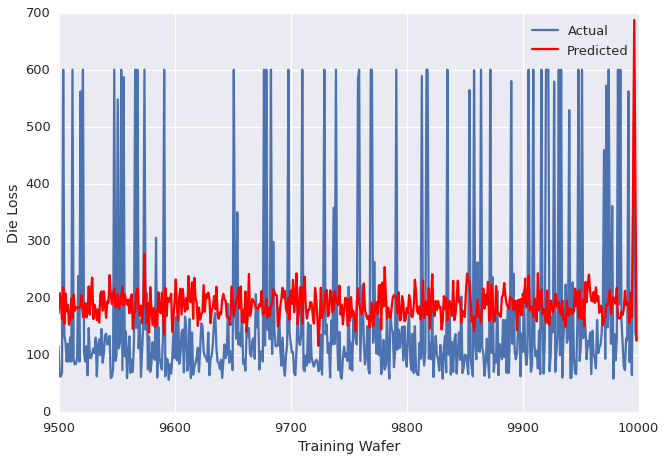### Ridge Model Full Prediction on the Final Validation Set¶

In :
Y_val_final = ridge_final.predict(X_val_final)
Y_val_final[Y_val_final > 600.0] = 600.0
Y_val_final[Y_val_final < 0.0] = 0.0
plt.plot(Y_val_final)
plt.xlabel("Validation Wafer")
_ = plt.ylabel("Predicted Die Loss")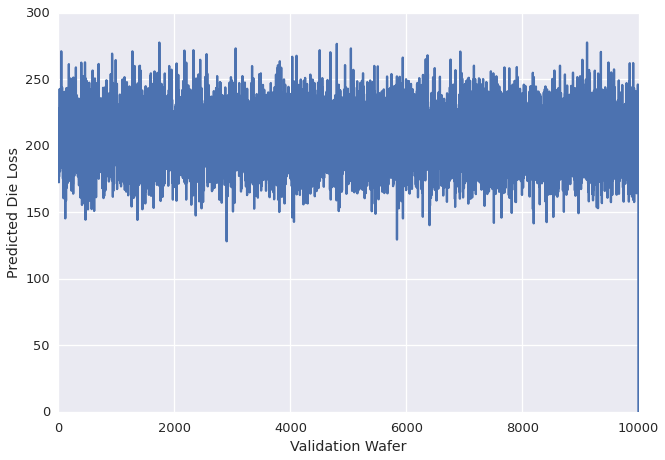## Predict A Constant for All Inputs¶

The Ridge model looks to be producing little more than a noisy constant as its prediction. What happens if we predict a noiseless constant near the Ridge prediction? The prediction below produces a score on the public leaderboard of 313.35.

In :
Y_val_final = 180.0 * np.ones(10000)


## Write Predictions for Submission¶

In :
np.savetxt('Y_val_final.csv', np.int32(Y_val_final),'%0.0i')